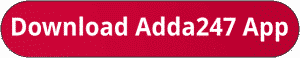Latest Banking jobs   »   IBPS RRB quant

# Quantitative Aptitude Quiz For IBPS RRB PO/Clerk Prelims 2023 -03rd July

Directions (1-5):- In each of the following questions, two equations (I) and (II) are given. Solve the equations and mark the correct option:
(a) if x>y
(b) if x≥y
(c) if x<y
(d) if x ≤y
(e) if x = y or no relation can be established between x and y.Direction (6-10): In each of the following questions, two equations (I) and (II) are given. Solve the equations and mark the correct option: (a) x>y
(b) y> x
(c) x ≥y
(d) x ≤y
(e) x =y or No relation can’t be established.Solutions. .                                                                                           .                                                                                                                                                               .                                                                               .

## FAQs

### What is the selection process of IBPS RRP PO?

The Selection Process of IBPS RRB PO consists of Prelims, Mains and Interview

#### Congratulations!Union Budget 2023-24: Free PDF